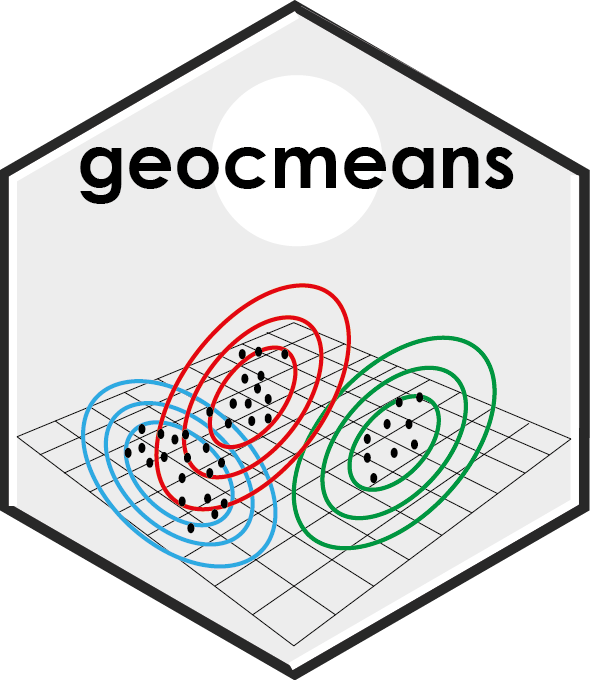# geocmeans## Installation

The stable version of `geocmeans` is available on CRAN. You can install it with the command below.

``install.packages("geocmeans")``

You can install a development version of the `geocmeans` package using the command below.

``remotes::install_github(repo = "JeremyGelb/geocmeans", build_vignettes = TRUE, force = TRUE)``

## Authors

Jeremy Gelb, Laboratoire d’Équité Environnemental INRS (CANADA), Email: jeremy.gelb@ucs.inrs.ca

## Contributors

Philippe Apparicio, Laboratoire d’Équité Environnemental INRS (CANADA), Email: philippe.apparicio@ucs.inrs.ca

Provides functions to apply Spatial Fuzzy c-means Algorithm, visualize and interpret results. This method is well suited when the user wants to analyze data with a fuzzy clustering algorithm and to account for the spatial dimension of the dataset. In addition, indexes for measuring the spatial consistency and classification quality are proposed. The algorithms were developed first for brain imagery as described in the articles of Cai and al. 2007 and Zaho and al. 2013. Gelb and Apparicio proposed to apply the method to perform a socio-residential and environmental taxonomy in Lyon (France). The methods can be applied to dataframes or to rasters.

#### Fuzzy classification algorithms

Four Fuzzy classification algorithms are proposed :

• FCM: Fuzzy C-Means, with the function `CMeans`
• GFCM: Generalized Fuzzy C-Means, with the function `GFCMeans`
• SFCM: Spatial Fuzzy C-Means, with the function `SFCMeans`
• SGFCM: Spatial Generalized Fuzzy C-Means, with the function `SGFCMeans`

Each function return a membership matrix, the data used for the classification (scaled if required) and the centers of the clusters.

#### Parameter selections

The algorithms available require different parameters to be fixed by the user. The function `selectParameters` is a useful tool to compare the results of different combinations of parameters. A multicore version, `selectParameters.mc`, using a plan from the package `future` is also available to speed up the calculus.

#### Classification quality

Many indices of classification quality can be calculated with the function `calcqualityIndexes`:

• Silhouette.index: the silhouette index (`fclust::SIL.F`)
• Partition.entropy: the partition entropy index (`fclust::PE`)
• Partition.coeff: the partition entropy coefficient (`fclust::PC`)
• Modified.partition.coeff: the modified partition entropy coefficient (`fclust::MPC`)
• XieBeni.index: the Xie and Beni index (`fclust::XB`)
• FukuyamaSugeno.index: the Fukuyama and Sugeno index (`geocmeans::calcFukuyamaSugeno`)
• DavidBoudlin.index: the David-Bouldin index (`geocmeans::calcDavidBouldin`)
• CalinskiHarabasz.index: the Calinski-Harabasz index (`geocmeans::calcCalinskiHarabasz`)
• GD43.index and GD53.index: two version of the generalized Dunn index (`geocmeans::calcGD43` and `calcGD53`)
• Negentropy.index: the Negentropy Increment index (`geocmeans::calcNegentropyI`)
• Explained.inertia: the percentage of total inertia explained by the solution

#### Classification consistency

To assess the stability of the obtained clusters, a function for bootstrap validation is proposed: `boot_group_validation`. The results can be used to verify if the obtained clusters are stable and how much their centres vary.

#### Interpretation

Several functions are also available to facilitate the interpretation of the classification:

• summary statistics for each cluster: `summarizeClusters` (also accessible with the generic function `summary`)
• spider charts: `spiderPlots`
• violin plots: `violinPlots`
• maps of the membership matrix: `mapClusters` (support polygon, points and polylines)

There is also a shiny app that can be used to go deeper in the result interpretation. It requires the packages `shiny`, `leaflet`, `bslib`, `plotly`, `shinyWidgets`, `car`.

#### Spatial diagnostic

Several spatial indices can be calculated to have a better spatial understanding of the obtained clusters, like the global or local Moran I calculated on the membership values, or the join-count-test on the most likely group for each observation. ELSA and Fuzzy ELSA statistics can also be calculated to identify areas with high or low multidimensional spatial autocorrelation in the membership values. See functions `spConsistency`, `calcELSA`, `calcFuzzyELSA` and `spatialDiag`.

We proposed an index to quantify the spatial inconsistency of a classification (Gelb and Apparicio). If in a classification close observations tend to belong to the same group, then the value of the index is close to 0. If the index is close to 1, then the belonging to groups is randomly distributed in space. A value higher than one can happen in the case of negative spatial autocorrelation. The index is described in the vignette `adjustinconsistency`. The function `spatialDiag` does a complete spatial diagnostic of the membership matrix resulting from a classification.

## Examples

Detailed examples are given in the vignette `introduction`

``vignette("introduction","geocmeans")``

## Testing

If you would like to install and run the unit tests interactively, include `INSTALL_opts = "--install-tests"` in the installation code.

``````remotes::install_github(repo = "JeremyGelb/geocmeans", build_vignettes = TRUE, force = TRUE, INSTALL_opts = "--install-tests")
testthat::test_package("geocmeans", reporter = "stop")``````

## Contribute

To contribute to `geocmeans`, please follow these guidelines.

Please note that the `geocmeans` project is released with a Contributor Code of Conduct. By contributing to this project, you agree to abide by its terms.

`geocmeans` version 0.1.0 is licensed under GPL2 License.